# IBPS CLERK MAINS 2017 – QUANTITATIVE APTITUDE DAY 15

Dear Banking Aspirants,

IBPS CLERK Mains 2017, the last exam of the year 2017 is going to held in 21.01.2018.There is only few days in our hands for preparation and you have to work hard to achieve your goal, because the competition is very high as per the vacancy is concerned.

So start your preparation now with this study Planner for IBPS Clerk Mains. It’s a 20 days complete planner with various Aptitude, Reasoning, English, Current affairs & Static Gk topics Included. There are 4 Mock tests available in this planner.

We have planned this Planner in Such a way that this will cover the entire current affairs quiz from the month of October to January (1st week). Because Banking Awareness, Current Affairs, Static Gk & Computer Awareness will play a vital role in cracking the IBPS Clerk Mains exam. Though the exam is getting tougher and tougher for each and every Year, Only consistently hard working candidates will crack the exam. So be a continuous hard worker and grab the opportunity to achieve your success.

### Make hay while the Sunshine!!!!!!!!!!!

So as per the planner here is content related to Quantitative Aptitude .

IBPS CLERK Mains 2017 -Quantitative Aptitude DAY 15

Quantitative Aptitude

Time: 20 Minutes

Topic: Time & work/time & distance

Q.1) Sheeja invested some amount at the rate of 6% pa simple interest and a certain sum at 10% p.a. SI. She received a yearly interest of Rs 1380. But if she had interchanged the amounts invested, she would have received Rs 40 less as interest. How much did she invest at 10%?

RS 8000

RS 8900

RS900

RS 9000

RS 12000

Q.2) What will be the ratio of SI earned by certain sum at the same rate of interest for 10 years and that for 12 years?

4:5

5:6

6:7

7:8

NONE OF THESE

Q.3) Raja lent Rs X to Vijay; Rs ‘Y’ to Kannan; Rs ‘Z’ to Vicky; He earned Rs ‘X’ as the simple interest for the amount lent to Kannan and Rs Y as the simple interest for the amount lent to Vicky. Find the relation among X, Y and Z assuming that the rate of interest and the period is uniform

Z=XY

X=YZ

Z^2=XY

Y=X^2 Z

Y^2=XZ

Q.4) Ranjana invested Rs 3200 at 12.5% p.a. simple interest for certain years. She got atotal amount of Rs 5600. Find number of years the sum is invested

4

9

8

6

7

Q.5) Urvashree invested a certain sum at 17.5% p.a.SI for 12 years. She got a total amount of Rs 17360.Find the sum.

RS 5600

RS 4600

RS 5000

RS 2300

RS 3400

Q.6) Maha invested a certain sum at 12% p.a.SI for 5 years and he invested 2/3rd of the sum at 8% for 12 years. He got a total interest of Rs 4464. Find the sum which is invested at 12%

RS 3000

RS 3600

RS 4300

RS 4600

RS 6000

Q.7) Vikram lent Rs 1300 at R1% p.a. SI for 6 years to Vijay and he lent Rs 6500 at R2% pa SI for 6 years to Siva. He earned a total interest of Rs 2300.Find R1

4%

5%

8%

7%

CAN’T BE DETERMINED

Q.8) Murugan invested a certain sum at 12% p.a. SI for 4 years and he invested 3/5th of the sum at 13% p.a. SI for 5 years. He got a total interest of Rs 4350. After that he invested the total amount in Scheme C which offers SI at 8% pa for 5 years. Find the interest earned by Murugan from scheme C?

RS 4980

RS 4890

RS 4940

RS 5480

NONE OF THESE

Q.9) Raja invested Rs 34000 at R% p.a. simple interest for 6 years. After 6 years he invested the total amount at 12% p.a. simple interest for 3 years. Finally he got the total amount of Rs 68435.2. Find the Rate of interest?

8%

4%

5%

9%

3%

Q.10) Nivetha invested Rs 3500 at 8% p.a. SI for 9 years. After 9 years She invested total amount at R% SI p.a. for 5 years. Finally she got a total amount of Rs 9030. Find the Rate (R%)

19%

12%

10%

8%

5%

Q.11) Walking at two-three of his usual speed, a man covers a certain distance in 3 hours more than the time he takes to cover the distance at his usual speed. The time taken by him to cover the same distance with his usual speed is same as the time taken by a woman to cover the distance of 300 km by motorbike. What is the speed of the motorbike?

50 KM/HR

45 KM/HR

40 KM/HR

55 KM/HR

60 KM/HR

Q.12) Ram takes 7 hours 15 minutes in walking a distance and riding back to the starting place. He could walk both ways in 8 hours 45 minutes. The time taken by him to ride both ways is 45 minutes more than the time will Gony take to drive both ways. Gony can walk a certain distance and drive back in 8 hours. How much time will he take to walk both ways?

13 HOURS

11 HOURS

14 HOURS

15 HOURS

9 HOURS

Q.13) A rat takes 6 leaps for every 5 leaps of cat, but 4 leaps of the cat are equal to 5 leaps of the rat. The ratio of the speed of the rat to that of cat is same as the minimum ratio of the speeds of train A and train B.The difference between the speeds of the two trains is 3 km/hr, and then what is the speed of train A?

72 KM/HR

48 KM/HR

108 KM/HR

120 KM/HR

NONE OF THESE

Q.14) A man perform a journey of 850 km partly by bus and partly by train. He takes 12 hours if he travels 250 km by bus and rest by car. He saves 2 hours, if he travels  the entire distance by car. The speed of the car is 35 km/hr more than the speed of the van. The van covers a distance of 400 km in what time?

8 HOURS

10 HOURS

12 HOURS

16 HOURS

15 HOURS

Q.15) kapoor covers a distance of 45 km at the speed of 9 km/hr and a distance of 24 km at the speed of 8km/hr. Further he covers a distance of 48 km at the speed of 12 km/hr. The average speed of kapoor is same as the average speed of Rahul. What is the total time taken by Rahul to cover the distance of 195 km in?

30 HOURS

25 HOURS

20 HOURS

15 HOURS

10 HOURS

Q.1) d

6x+10y=138000 —- (1)

6y+10x=134000 —- (2)

By solving above 2 equation we get y=Rs 9000

Q.2) Required ratio=10:12=5:6

Q.3) e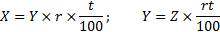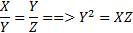Q.4) d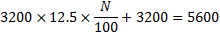N=6

Q.5) a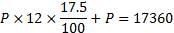P=Rs 5600

Q.6) b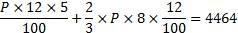P=3600

Q.7) e

Can’t be determined as data inadequate

Q.8) c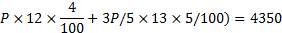P=5000

Total=5000+3000+4350=12350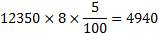Q.9) b

From case II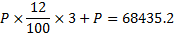P=50320

To find the value of R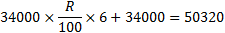R=8%

Q.10)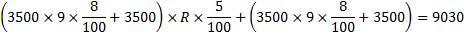R=10%

Q.11) a

Walking at 2/3rd of his usual speed, he will take 3/2nd of usual time.

3/2 of the usual time = usual time + 3 hours

½ of the usual time = 3 hours.

Usual time = 2×3 = 6 hours.

Required motor bike speed = 300/6=50km/hr

Q.12) b

One way walking + one way riding = 7 hours 15 minutes.

2×(one way walking +one way riding) = 14 hours 30 minutes.

Two ways walking time = 8 hours 45 minutes.

Two ways riding time  = 14 hours 30 minutes – 8 hours 45 minutes = 5 hours 45 minutes

Gony’s two way driving time = 5 hours 45 minutes-45 minutes = 5 hours.

One way walking + one way riding = 8 hours

2×(one way walking +one way riding) = 16 hours

Two ways walking time = 16-5 = 11 hours

Q.13) a

Rat              Cat

Frequency of leaps          =       6                 5

Ratio of length of leaps   =       4                 5

Speed of rat: Speed of cat = 6×4: 5×5 = 24: 25

Required speed,

x= 3

The speed of train A = 72 km/hr.

Q.14) a

Time taken to cover the whole journey by the car = 12-2 = 10 hours

Speed of the car = 850/10 = 85 km/hr

The speed of the van = 85-35 = 50 km/hr

Required time = 400/50 = 8hours.

Q.15) c

Total distance covered by kapoor = 45+ 24+ 48 = 117 km

Total time taken by kapoor = 45/9+24/8+48/12=12 hours

Average speed of kapoor = 117/12 = 9.75 km/hr

Time taken by Rahul = 195/9.75=20 hours

You can join in our Telegram Channel the invite given below and you can share this with your friends and other aspirants who are preparing for their exams like you.

Caution: You should have Telegram app installed in your mobile or laptop to join the group. If you don’t have telegram app in your mobile. First install the app and click the link given below….